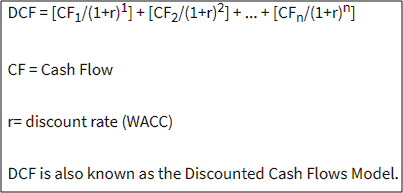How to value stocks using discounted cash flow method - Motilal Oswal
How to value stocks using discounted cash flow method - Motilal Oswal

# How to value stocks using discounted cash flow methodWhy do we value stocks? The answer is that valuing stocks helps us to know whether the market is undervaluing the stock or overvaluing the stock. Your decision to buy or sell the stock will be based on this input. There are many methods of valuing a company but one of the more popular methods is the Discounted Cash Flow method (DCF). The DCF valuation method is simple and elegant, as we shall see later. The DCF stock valuation calculator is available in many websites but it is perhaps more important to understand the gist of the concept of DCF. In a nutshell, the DCF method projects the cash flows for the future and then discounts them to the present to get a present value. This present value is an approximation of the valuation of the company, to which many qualitative adjustments will be required.

Key components of DCF valuation methodology..The formula above best captures the gist of DCF valuation methodology. Here are four key aspects of the formula that you need to understand.

The DCF method focuses on cash flows rather on profits. So, what do we understand by cash flows. You use the same formula as you use in your cash flow statement. You take the profit after tax and then add back depreciation, amortization and other non-cash charges. From this figure, you deduct the average annual capital expenditure that needs to be funded internally. The balance that you get is the yearly cash flows and is used as the key input for valuation purpose.

Projecting periodic cash flows..
Practically it is not possible to predict cash flows for 20 years so you should stick to projecting the cash flows for a maximum of next 5 years. In an uncertain business environment, that itself is hard enough. How do you project cash flows for the next five years? You start off with your own projections by extrapolating the data using statistical methods. Then data is ratified by applying industry level growth parameters. Subsequently, the management discussion and management guidance helps to understand the nuances involved in future year cash flows. Finally, inputs are also taken from competitors and dealers to get a clearer picture. It is based on all these inputs that the future cash flows are projected for the next five years.

Projecting the terminal cash flows..
What do we mean by terminal cash flows? When you buy an equity share it is a perpetual asset. That means it is assumed that the business continues forever. But projection of cash flows is feasible only for the next five years. At the end of the fifth year you assume that the company will maintain a perpetual growth rate from that point onwards. At the end of the fifth the present value of the perpetual cash flows will be calculated and that becomes the terminal cash flow at the end of the fifth year.

Discounting the future cash flows to the present..
So now you have projected cash flows for each of the next five years. Having done that, you have also calculated the terminal value of the company at the end of the fifth year. But all these are future cash flows and we are talking about valuation in the present. Therefore we have to calculate the present value of these cash flows. Let us dwell for a moment on the importance of present value.
We all know that money has a cost. So your Rs.100 today is worth Rs.110 at the end of 1 year and Rs.121 at the end of 2 years assuming 10% rate of interest. Looking at it the other way, the present value of Rs.110 at the end of 1 year is Rs.100 today and the present value of Rs.121 after 2 years is also Rs.100 today. When it comes to DCF, we use the cost of capital, which is the weighted average of the cost of equity and the cost of debt. The rate of discount represented by “r” in the above formula is the cost of capital which is used to discount the future cash flows backward.

Got the DCF valuation; is the job over?
So finally, you got the DCF valuation. Does that really complete your valuation job? Not exactly, because you still need to make adjustments to it to make the DCF meaningful..

Remember, DCF works best in case of companies that have stable business models and are growing at a stable rate. Start-up firms, companies requiring huge capital outlays, companies with long gestation for profits cannot use the DCF method as it will distort the valuations.

The DCF valuation once calculated needs to be mugged with realism. If you get a valuation of 25X for a metals company, there is obviously something off the mark. You need to look at domestic and global benchmarks of P/E ratio and P/BV ratio to make a more reasonable call o the DCF valuation.

Finally, you need to understand margin of safety when it comes to DCF valuation. DCF is very data sensitive. A change in the growth or the cost of capital can make a huge impact n valuation. Hence it is safer to keep a margin of safety. For example, you can stipulate that a stock is underpriced only if the market price is at least 20% below the DCF valuation. That will give you a margin of safety and reduce your overall risk.

Many critics call DCF rear-view mirror valuation. But it is among the best working models available. As an investor you need to make the best of this method to get a hang of the stock.

## Ready to invest with us?

Share your Mobile Number with us and get started

• +91|